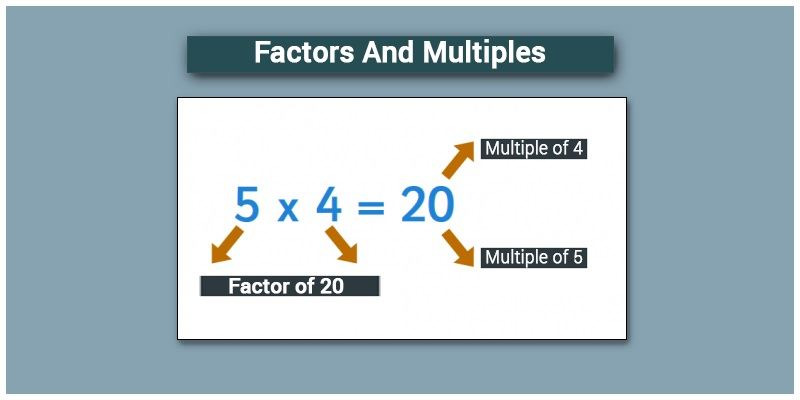# Factors And Multiples

In lower classes, we have learnt multiplication table for numbers. It is time to recollect, to understand the concept of multiples and factors of a number.

FACTORS

When a number is said to be a factor of any other second number, then the first number must divide the second number completely without leaving any remainder. In simple words, if a number (dividend) is exactly divisible by any number (divisor), then the divisor is a factor of that dividend. Every number has a common factor that is one and the number itself.For example, 4 is a factor of 24 i.e. 4 divides 24 exactly giving 6 as quotient and leaving zero as remainder. Alternatively, 6 is also a factor of 24 as it gives 4 as quotient on division. Therefore, 24 has 1, 24, 4, 6 as its factors in addition to 2, 3, 8 and 12 and all these numbers divide 24 exactly leaving no remainder.

If any natural number has only two factors i.e. 1 and the number itself as its factors, such numbers are called prime numbers. 2 is an example of prime number where it has only two factors 1 and 2.

MULTIPLES

A multiple of a number is a number that is the product of a given number and some other natural number. Multiples can be observed in a multiplication table. Multiples of some numbers are as follows:

Multiples of 2 are

2 × 1 = 2,

2 × 2 = 4,

2 × 3 = 6,

2 × 4 = 8,

2 × 5 = 10,

2 × 6 = 12,

2 × 7 = 14,

2 × 8 = 16,

and so on. Hence, multiples of 2 will be even numbers and will end with 0, 2, 4, 6 or 8.

Multiples of 3 are

3 × 1 = 3,

3 × 2= 6,

3 × 3 = 9,

3 × 4 = 12,

3 × 5= 15,

3 × 6 = 18

and so on.

Multiples of 5 are

5 × 1 = 5,

5 × 2 = 10,

5 × 3 = 15,

5 × 4 = 20,

5 × 5 = 25,

and so on. Every multiple of 5 has its last digit as 0 or 5.

FACTOR vs MULTIPLE

Some may get confused with the concept of multiple and factor. Let us consider an example. In the multiplication table of 6, we have 6 x 3 = 18. Here, 6 and 3 are the factors of 18 while 18 is the multiple of 3 as well as 6. This means both 6 and 3 divides 18 which is same as that 18 is exactly divisible by 6 and 3.

Thus, we can conclude that if X and Y are two numbers and;

•    If X divides Y, X is a factor of Y

•    If Y is divisible by X, Y is a multiple of X

Since the number 1 divides every integer, it is a common factor of every integer. Also, every number is divisible by 1 and every number is a multiple of 1.

To solve problems on the topic, download Byju’s-The Learning app from Google Play Store and watch interactive videos. Also, take free tests to practice for exams. To study about other topics, visit http://www.byjus.com/ and browse among thousands of interesting articles.